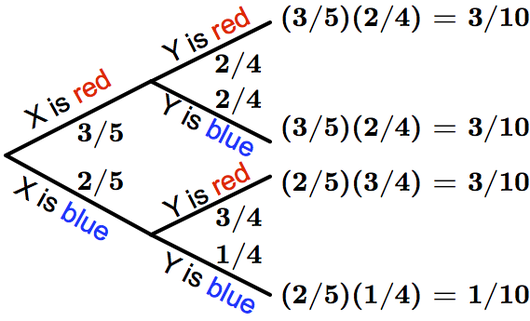# Glossary

## Dependent random variables

Two random variables are called "dependent" if the probability of events associated with one variable influence the distribution of probabilities of the other variable, and vice-versa. The word "influence" is somewhat misleading, as causation is not a necessary component of dependence.

For example, consider drawing two balls from a hat containing three red balls and two blue balls. If $X$ is the random variable associated with the color of the first ball, and $Y$ is the color of the second ball, then clearly the value of $X$ and $Y$ will influence each other: if we draw a red ball first, then the probabilities for $Y$ are different than what they would be if we draw a blue ball first. Below is the probability tree diagram for the two drawings.There are two ways of stating dependence mathematically. First, we might say that $X$ and $Y$ are dependent if they are not independent. In other words, there exist events $A$ and $B$ containing outcomes of $X$ and $Y$, respectively, such that $\mathrm{Pr}(A \textrm{ and } B)$ is not equal to $\mathrm{Pr}(A) \times \mathrm{Pr}(B)$. In the case of drawing balls, let $A$ be the outcome of the first ball drawn being blue, and $B$ be the outcome of the second ball drawn being blue. Then $\mathrm{Pr}(A \textrm{ and } B)$ is 1/10, although $\mathrm{Pr}(A)$ is 2/5 and $\mathrm{Pr}(B)$ is 2/5.

An equivalent way of saying that $X$ and $Y$ are dependent is if there are events $A$ and $B$ containing respective outcomes corresponding to $X$ and $Y$ such that the conditional probability $\mathrm{Pr}(A \mid B)$ is not equal to $\mathrm{Pr}(A)$. Using the same events for our example variables $X$ and $Y$ above, we can see that while $\mathrm{Pr}(A \mid B)$ is 2/5, $\mathrm{Pr}(A \mid B)$ is $\mathrm{Pr}(A \textrm{ and } B)/\mathrm{Pr}(B)$, or 1/4. This calculation makes sense, since if we know that the second ball drawn is blue, odds are much better that the first ball was red than blue.